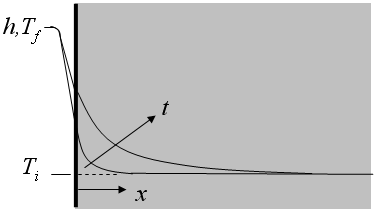Contents - Index

ConvectionFunction semiinf3(T_i,T_f,h,k,alpha,x,time) returns the temperature for a given position and time within a semi-infinite body with surface convection using the relation provided in Table 3-2 of Nellis and Klein.

Inputs:
T_i - initial temperature of the solid  [C] or [K]
T_f - bulk fluid temperature [C] or [K]
h - convection coefficient [W/m^2-K]
alpha - thermal diffusivity [m^2/s]
x - perpendicular distance from surface [m]
time - time relative to surface exposure (surface convection begins at t=0) [s]

This function can be used with English units set in EES.  In this case, T_i and T_f are in [F] or [R], h is in Btu/hr-ft^2-R, alpha is in ft^2/hr and x is in [ft].  Time must be in s.

Example:
\$UnitSystem SI K Pa J
T_i=393[K]
T_f=355 [K]
h = 50 [W/m^2-K]
alpha=0.000004029 [m^2/s]
k=10 [W/m-K]
x=0.003 [m]
time=100 [s]
T=semiinf3(T_i,T_f,h,k,alpha,x,time)

{Solution: T=389.5 [K]}

Transient Conduction Index